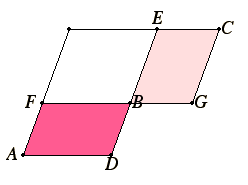# Proposition 14

In equal and equiangular parallelograms the sides about the equal angles are reciprocally proportional; and equiangular parallelograms in which the sides about the equal angles are reciprocally proportional are equal.
I.14

Let AB and BC be equal and equiangular parallelograms having the angles at B equal, and let DB and BE be placed in a straight line. Therefore FB and BG are also in a straight line.

I say that, in AB and BC, the sides about the equal angles are reciprocally proportional, that is to say, DB is to BE as BG is to BF.I.31

Complete the parallelogram FE.

V.7

Then since the parallelogram AB equals the parallelogram BC, and FE is another parallelogram, therefore AB is to FE as BC is to FE.

But AB is to FE as DB is to BE, and BC is to FE as BG is to BF. Therefore DB is to BE as BG is to BF.

Therefore in the parallelograms AB and BC the sides about the equal angles are reciprocally proportional.

Next, let DB be to BE as BG is to BF.

I say that the parallelogram AB equals the parallelogram BC.

Since DB is to BE as BG is to BF, while DB is to BE as the parallelogram AB is to the parallelogram FE, and, BG is to BF as the parallelogram BC is to the parallelogram FE, therefore also AB is to FE as BC is to FE.

V.9

Therefore the parallelogram AB equals the parallelogram BC.

Therefore, in equal and equiangular parallelograms the sides about the equal angles are reciprocally proportional; and equiangular parallelograms in which the sides about the equal angles are reciprocally proportional are equal.

Q.E.D.

## Guide

This proposition is used in the proofs of propositions VI.16, VI.30, and X.22.